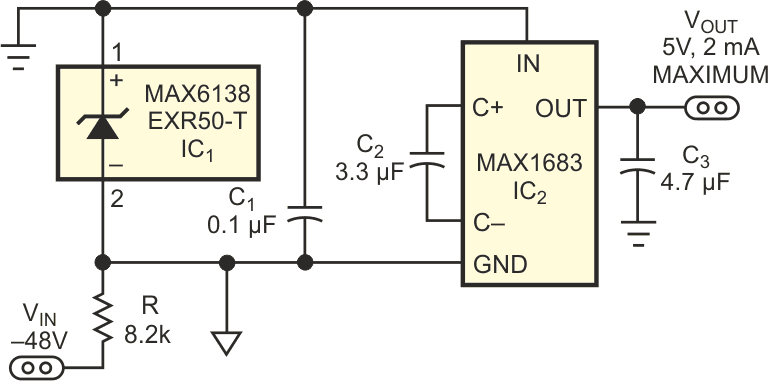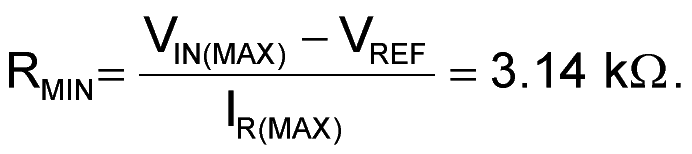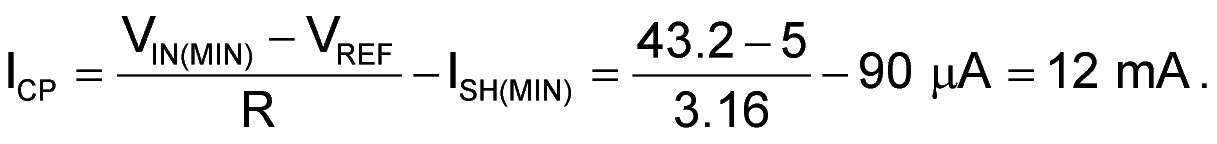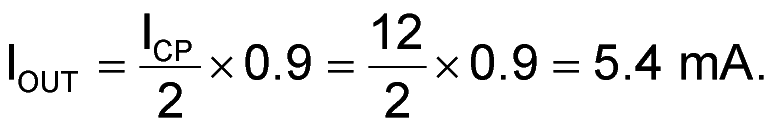# Simple circuit provides 5V gate bias from -48V

## Maxim MAX6138 MAX1683

EDN

A small and simple circuit derives 5 V from the –48 V rail that telecomm applications typically use (Figure 1). Useful for gate bias and other purposes, the 5 V supply delivers as much as 5-mA output current. A shunt reference, IC1, defines –5 V as ground reference for a charge pump, IC2. The charge pump doubles this 5 V difference between system ground and charge-pump ground to produce 5 V with respect to the system ground. The shunt reference maintains 5 V across its terminals by regulating its own current, IS. IS is a function of the value of R. The current through R, IR, is reasonably constant and varies only with the input voltage. IR, the sum of the charge-pump and shunt-reference currents (IR = ICP + IS), has maximum and minimum values set by the shunt reference.Figure 1. This small, simple circuit produces 5 V at 5 mA from a –48 V input.

The shunt reference sinks as much as 15 mA and requires 60 µA minimum to maintain regulation. Maximum IR is a function of the maximum input voltage. To prevent excessive current in the shunt reference with no load on the charge-pump output, use the maximum input voltage (–48 V –10% = –52.8 V) to calculate the minimum value of R. The maximum reference sink current, 15 mA, plus the charge pump's no-load operating current, 230 µA, equals the maximum IR value, 15.23 mA. Thus,Choose the next-highest standard 1% value, which is 3.16 kΩ. You calculate the guaranteed output current for the charge pump at the minimum line voltage: –48 V + 10% = –43.2 V. The charge pump's maximum input current iswhere 90 µA is the minimum recommended operating current for the shunt reference. Assuming 90% efficiency in the charge pump, the output current isYou halve the charge-pump current, because the output voltage is twice the input voltage. Be sure that R can handle the wattage under no-load conditions. A 1 W resistor suffices in this example.

EDN